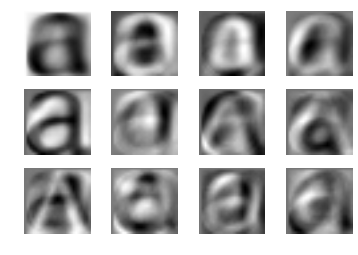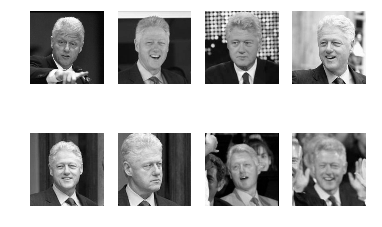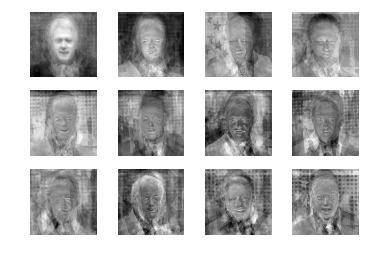# Applying PCA Analysis on Images

Image_PCA_analysis

# Apply PCA analysis on Images¶

## To extract the main characteristics for a group of images¶

##### Better apply PCA to extracted features¶
In :
import osimport globimport mathfrom PIL import Imageimport numpy as npimport matplotlib.pyplot as plt# Ensure plots embeded in notebook%matplotlib inline
In :
# Prepare data# In this case, font images for "a"imgSrcDir = 'a_font_thumbs'a_font_files = [os.path.join(imgSrcDir, f) for f in os.listdir(imgSrcDir) if f.endswith(".jpg")]
In :
# Define PCA functiondef pca(X):    """    Principal Component Analysis    input: X, matrix with trainnig data stored as flattened arrays in rows    return: projection matrix (with important dimensions first), variance and mean.        SVD factorization:  A = U * Sigma * V.T                        A.T * A = V * Sigma^2 * V.T  (V is eigenvectors of A.T*A)                        A * A.T = U * Sigma^2 * U.T  (U is eigenvectors of A * A.T)                        A.T * U = V * Sigma                            """        # get matrix dimensions    num_data, dim = X.shape        # center data    mean_X = X.mean(axis=0)    X = X - mean_X        if dim > num_data:        # PCA compact trick        M = np.dot(X, X.T) # covariance matrix        e, U = np.linalg.eigh(M) # calculate eigenvalues an deigenvectors        tmp = np.dot(X.T, U).T        V = tmp[::-1] # reverse since the last eigenvectors are the ones we want        S = np.sqrt(e)[::-1] #reverse since the last eigenvalues are in increasing order        for i in range(V.shape):            V[:,i] /= S    else:        # normal PCA, SVD method        U,S,V = np.linalg.svd(X)        V = V[:num_data] # only makes sense to return the first num_data    return V, S, mean_X
In :
# load images into matriximmatrix = np.array([np.array(Image.open(im, 'r')).flatten()                 for im in a_font_files], 'f')
In :
# Perform PCAV, S, immean = pca(immatrix)
Path b
In :
# Show Results# First one is the mean image# Rest 7 are the top 7 features extracted for font 'a'tmp_img = np.array(Image.open(a_font_files, 'r'))m,n = tmp_img.shapefig = plt.figure()plt.gray()plt.subplot(3,4,1)plt.imshow(immean.reshape(m,n))plt.axis('off')for i in range(11):    plt.subplot(3,4,i+2)    plt.imshow(V[i].reshape(m,n))    plt.axis('off')plt.show()### Apply PCA to Face Images :-)¶

In :
## List of Bill Clinton Face Imageslwf_src_dir = "lfw/Bill_Clinton"bclinton_files = [os.path.join(lwf_src_dir, f) for f in os.listdir(lwf_src_dir) if f.endswith(".jpg")]## Display first 7 imagesfig_org = plt.figure()tmp_img = np.array(Image.open(bclinton_files, 'r').convert('L'),'f')m,n = tmp_img.shape# load images into matrixlwf_immatrix = np.array([np.array(Image.open(im, 'r').convert('L'),'f').flatten()                 for im in bclinton_files], 'f')for i in range(8):    plt.subplot(2,4,i+1)    plt.imshow(lwf_immatrix[i].reshape(m,n))    plt.axis('off')In :
# PCA on face imagesbV, bS, bimmean = pca(lwf_immatrix)
In :
# Show Results# First one is the mean image# Rest 11 are the top 11 features extracted for pictures of Bill Clintonfig = plt.figure()#plt.gray()plt.subplot(3,4,1)plt.imshow(bimmean.reshape(m,n))plt.axis('off')for i in range(11):    plt.subplot(3,4,i+2)    plt.imshow(bV[i].reshape(m,n))    plt.axis('off')plt.show()In [ ]:


Published: September 07 2016

• category:
• tags: